Sie sind hier: ICP » R. Hilfer » Publikationen

# 3 Time Evolution of Observables

[209.2.1] Time is commonly considered as the set of Aristotelian time instants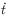. [209.2.2] The set of all time instants is represented mathematically by the set of real numbers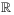. [209.2.3] Time is “measured” by observing clocks. [209.2.4] Clocks are physical systems. [209.2.5] Let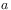be an observable quantity (e.g. the position of the sun, the moon or some hand on a watch), and let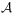be the set of observables of such a physical system. [209.2.6] A dynamical system is a triple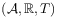whereis the set of observables of a physical system,represents time, and the mapping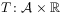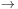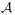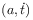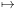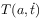(1)

is its dynamical rule . [209.2.7] It describes the change of observable quantities with time. [209.2.8] For the dynamical rule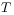the following properties are postulated:

1. [209.2.9] For all time instants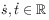the dynamical rule obeys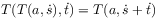(2)

for all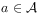.

2. [209.2.10] There exists a time instant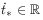, called beginning, such that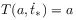(3)

holds for all.

3. [209.2.11] The mapis continuous in time in a suitable topology.

[209.2.12] The set of observables reflects the kinematical structure of the physical system. [209.2.13] The dynamical rule prescribes the time evolution of the system. [page 210, §0]    [210.0.1] Setting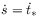in eq. (2) and using eq. (3) shows, that either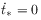must hold, or else the observable must be time independent. [210.0.2] The time evolution of observables is the one-parameter family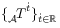of maps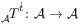defined by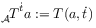(4)

for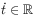. [210.0.3] The time evolution obeys the group law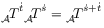(5)

for all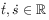, and the identity law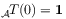(6)

where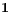is the identity on. [210.0.4] The continuity law requires a topology. [210.0.5] It is usually assumed, thatis a Banach space with norm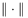, and that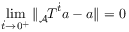(7)

holds for all. [210.0.6] Equations (5),(6) and (7) define a strongly continuous one parameter group of operatorson, called a flow [26, 27]. [210.0.7] For bounded linear operators strong and weak continuity are equivalent .

[210.1.1] Identifying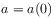and writing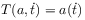the time evolution becomes time translation to the left, i.e.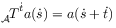(8)

for all. [210.1.2] If the arrow of time is taken into account, then the flow of time is directed, and only the time instants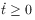after the beginning can occur. [210.1.3] In that case, inverse elements do not exist, and the family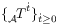of operators forms only a semigroup [29, 28] instead of a group.